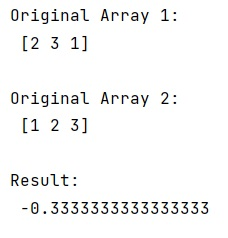# Calculating Covariance using numpy.cov() method

Learn, how to calculate the covariance using numpy.cov() method in Python?
Submitted by Pranit Sharma, on January 18, 2023

NumPy is an abbreviated form of Numerical Python. It is used for different types of scientific operations in python. Numpy is a vast library in python which is used for almost every kind of scientific or mathematical operation. It is itself an array which is a collection of various methods and functions for processing the arrays.

## Python - numpy.cov() Method

In mathematics and statistics, covariance is a measure of the relationship between two random variables. The metric evaluates how much – to what extent – the variables change together. In other words, it is essentially a measure of the variance between two variables.

We can also understand covariance as a value that indicates the level to which two variables vary together. If we examine N-dimensional samples, X=[x1,x2,...xn]T, then the covariance matrix element Cij is the covariance of xi and xj The element Cii is the variance of xi.

Numpy provides us a method i.e., numpy.cov(). If we have two 1-dimensional numpy arrays and we pass them in this method as an argument, it calculates their covariance.

Let us understand with the help of an example,

## Python code to calculate the covariance using numpy.cov() method

```# Import numpy
import numpy as np

# Creating two numpy arrays
arr1 = np.array([2, 3, 1])
arr2 = np.array([1,2,3])

# Display original arrays
print("Original Array 1:\n",arr1,"\n")
print("Original Array 2:\n",arr2,"\n")

# Getting covariance
res = np.cov(arr1, arr2, bias=True)

# Display result
print("Result:\n",res)
```

Output:What's New (MCQs)

Top Interview Coding Problems/Challenges!

IncludeHelp's Blogs

Languages: » C » C++ » C++ STL » Java » Data Structure » C#.Net » Android » Kotlin » SQL
Web Technologies: » PHP » Python » JavaScript » CSS » Ajax » Node.js » Web programming/HTML
Solved programs: » C » C++ » DS » Java » C#
Aptitude que. & ans.: » C » C++ » Java » DBMS
Interview que. & ans.: » C » Embedded C » Java » SEO » HR
CS Subjects: » CS Basics » O.S. » Networks » DBMS » Embedded Systems » Cloud Computing
» Machine learning » CS Organizations » Linux » DOS
More: » Articles » Puzzles » News/Updates